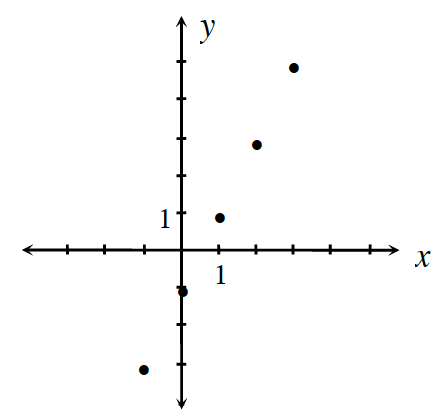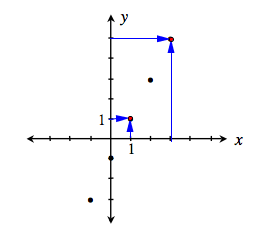### Home > CC3MN > Chapter 3 Unit 3 > Lesson CC3: 3.2.2 > Problem3-85

3-85.

Examine the graph at right.1. Use the graph to complete the table:

 IN (x) OUT (y)
 IN ($x$) $\color{blue}{1}$ $\color{blue}{3}$ OUT ($y$) $\color{blue}{1}$ $\color{blue}{5}$2. Use the graph to find the rule:
$y=$ ___________

The graph is a line, so use what you know about rules in tables.